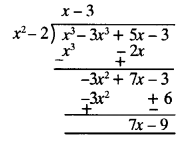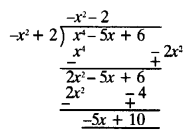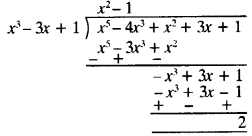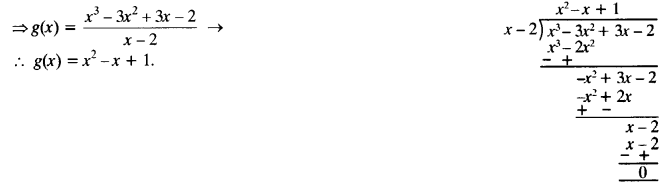# NCERT Solutions for Class 10 Maths Chapter 2 Polynomials Ex 2.3

## NCERT Solutions for Class 10 Maths Chapter 2 Polynomials Ex 2.3

Ex 2.3 Class 10 Question 1.
Divide the polynomial p(x) by the polynomial g(x) and find the quotient and remainder, in each of the following:
(i) p(x) = x3 – 3x2 + 5x -3, g(x) = x2-2
(ii) p(x) =x4 – 3x2 + 4x + 5, g(x) = x2 + 1 -x
(iii) p(x) = x4 – 5x + 6, g(x) = 2 -x2
Solution:
(i) Here p(x) = x3 -3x2 + 5x – 3 and g(x) = x2 -2
dividing p(x) by g(x) ⇒Quotient = x – 3, Remainder = 7x – 9

(ii) Here p(x) = x4– 3x2 + 4x + 5 and g(x) = x2 + 1 -x
dividing p(x) by g(x) ⇒Quotient = x2 + x – 3, Remainder = 8

(iii) Herep(x) = x4– 5x + 6 and g(x) = 2-x2
Rearranging g(x) = -x2 + 2
dividing p(x) by g(x) ⇒
Quotient = -x2 – 2
Remainder = -5x + 10.Ex 2.3 Class 10 Question 2.
Check whether the first polynomial is a factor of the second polynomial by dividing the second polynomial by the first polynomial:
(i) t2-3, 2t4 + t3 – 2t2 – 9t – 12
(ii) x2 + 3x + 1,3x4+5x3-7x2+2x + 2
(iii) x3 -3x + 1, x5 – 4x3 + x2 + 3x + l
Solution:
(i) First polynomial = t2 – 3,
Second polynomial = 2t4 + 3t3 – 2t2 – 9t – 12
dividing second polynomial
by first polynomial ⇒
∵ Remainder is zero.
∴First polynomial is a factor of second polynomial.(ii) First polynomial = x2 + 3x + 1
Second polynomial = 3x4 + 5x3 – 7x2 + 2x + 2
dividing second polynomial
by first polynomial ⇒
∵ Remainder is zero.
∴ First polynomial is a factor of second polynomial.(iii) First polynomial = x3 – 3x + 1
Second polynomial = x5 – 4x3 + x2 + 3x + 1.
∵ Remainder ≠ 0.
∴ First polynomial is not a factor of second polynomial.Ex 2.3 Solutions Class 10 Question 3.
Obtain all other zeroes of 3x4 + 6x3 – 2x2 – 10x – 5, if two of its zeroes are 53−−√ and –53−−√
Solution:NCERTSolutions Ex 2.3 Class 10 Question 4.
On dividing x3 – 3x2 + x + 2 by a polynomial g(x), the quotient and remainder were x – 2 and -2x + 4, respectively. Find g(x).
Solution:
p(x) = x3 – 3 x 2 + x + 2 g(x) = ?
Quotient = x – 2; Remainder = -2x + 4
On dividing p(x) by g(x), we have
p(x) = g(x) x quotient + remainder
⇒ x3– 3x2 + x + 2 = g(x) (x – 2) + (-2x + 4)
⇒ x– 3x2 + x + 2 + 2 x- 4 = g(x) x (x-2)
⇒ x3 – 3x2 + 3x – 2 = g(x) (x – 2)Exercise 2.3 Class 10 Maths Question 5.
Give examples of polynomials p(x), g(x), q(x) and r(x), which satisfy the division algorithm and
(i) deg p(x) = deg q(x)
(ii) deg q(x) = deg r(x)
(iii) deg r(x) = 0
Solution:
(i) p(x),g(x),q(x),r(x)
deg p(x) = deg q(x)
∴ both g(x) and r(x) are constant terms.
p(x) = 2x2– 2x + 14; g(x) = 2
q(x) = x2 – x + 7; r(x) = 0

(ii) deg q(x) = deg r(x)
∴ this is possible when
deg of both q(x) and r(x) should be less than p(x) and g(x).
p(x) = x3+ x2 + x + 1; g(x) = x2 – 1
q(x) = x + 1, r(x) = 2c + 2

(iii) deg r(x) is 0.
This is possible when product of q(x) and g(c) form a polynomial whose degree is equal to degree of p(x) and constant term.